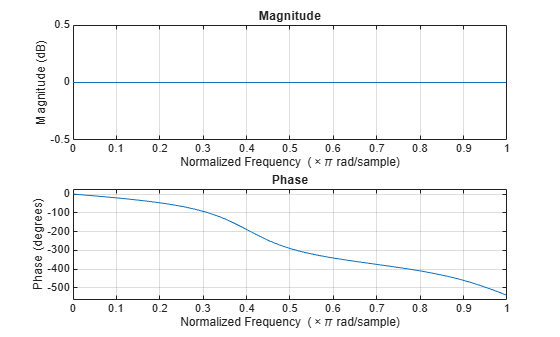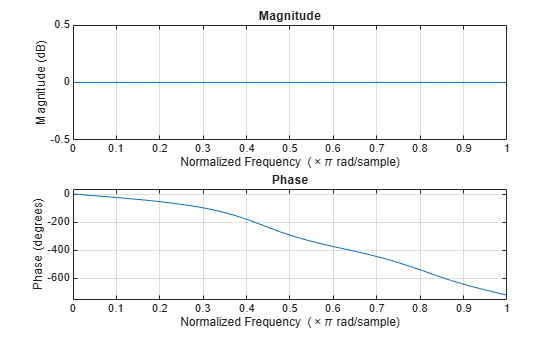Documentation

# isallpass

Determine whether filter is allpass

## Syntax

```flag = isallpass(b,a) flag = isallpass(sos) flag = isallpass(d) flag = isallpass(...,tol) flag = isallpass(hd) ```

## Description

`flag = isallpass(b,a)` returns a logical output, `flag`, equal to `true` if the filter specified by numerator coefficients, `b`, and denominator coefficients, `a`, is an allpass filter. If the filter is not an allpass filter, `flag` is equal to `false`.

`flag = isallpass(sos)` returns `true` if the filter specified by second order sections matrix, `sos`, is an allpass filter. `sos` is a K-by-6 matrix, where the number of sections, K, must be greater than or equal to 2. Each row of `sos` corresponds to the coefficients of a second order (biquad) filter. The ith row of the `sos` matrix corresponds to ```[bi(1) bi(2) bi(3) ai(1) ai(2) ai(3)]```.

`flag = isallpass(d)` returns `true` if the digital filter, `d`, is an allpass filter. Use `designfilt` to generate `d` based on frequency-response specifications.

`flag = isallpass(...,tol)` uses the tolerance, `tol`, to determine when two numbers are close enough to be considered equal. If not specified, `tol`, defaults to `eps^(2/3)`. Specifying a tolerance may be most helpful in fixed-point allpass filters.

```flag = isallpass(hd)``` returns `true` if the filter object `hd` is an allpass filter.

## Examples

collapse all

Create an allpass filter and verify that the frequency response is allpass.

```b = [1/3 1/4 1/5 1]; a = fliplr(b); flag = isallpass(b,a)```
```flag = logical 1 ```
`fvtool(b,a)`Create a lattice allpass filter and verify that the filter is allpass.

```k = [1/2 1/3 1/4 1/5]; [b,a] = latc2tf(k,'allpass'); flag_isallpass = isallpass(b,a)```
```flag_isallpass = logical 1 ```
`fvtool(b,a)`